Abbreviation for "top left": t-l (a triangle points to the top left) Abbreviation for "bottom right": b-r (a triangle points to the bottom right) Abbreviation for "in a full square": in square (the sign is inverse)

red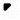triangle t-l
bright red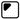triangle t-l, with frame
dark red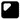triangle t-l, in square
yellow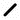slash
bright yellow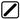slash, with frame
dark yellow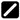slash, in square
blue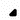triangle b-r
bright blue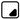triangle b-r, with frame
dark blue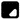triangle b-r, in square
orange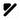triangle t-l, slash
bright orange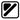triangle t-l, slash, with frame
dark orange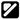triangle t-l, slash, in square
greenslash, triangle b-r
bright green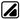slash, triangle b-r, with frame
dark green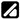slash, triangle b-r, in square
brown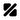triangle t-l, slash, triangle b-r
bright brown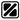triangle t-l, slash, triangle b-r, with frame
dark brown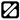triangle t-l, slash, triangle b-r, in square
violet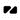triangle t-l, triangle b-r
bright violet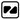triangle t-l, triangle b-r, with frame
dark violet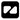triangle t-l, triangle b-r, in square
white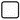empty frame
black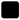full square
bright gray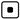dot, with frame
dark gray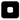dot, in square
silver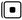dot, with frame, to the left of it a vertical line
gold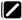slash, in square, to the left of it a vertical linemain page: fakoo.de/en/color